Create a new printable
Answer key also includes questions
Answer key only gives the answers
No answer keyMath Worksheets

Sample - Click above to make a new math worksheet (PDF).
 Name _____________________________Date ___________________
Subtraction and Addition
Subtract and then add to check your answer.

1.
What is 68 - 9?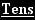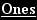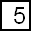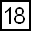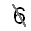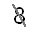- 9 5 9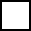9 +6 8
2.
What is 92 - 3?9 2 - 33 +9 2
3.
What is 27 - 8?2 7 - 88 +2 7
 4 * This is a pre-made sheet.Use the link at the top of the page for a printable page.
5.
What is 46 - 8?4 6 - 88 +4 6
6.
What is 80 - 7?8 0 - 77 +8 0

 Answer Key

Sample
This is only a sample worksheet.

Create a new printable
Answer key also includes questions
Answer key only gives the answers
No answer keyMath Worksheets